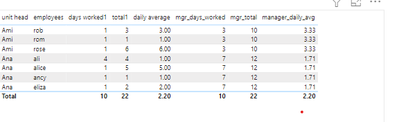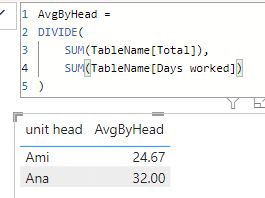cancel
Showing results for
Did you mean:Frequent Visitor

 I am a newbie in power Bi unit head employees Days worked Total Daily Average Ana alice 2 88 44 eliza 2 40 20 ancy 1 4 2 ali 3 124 62 Ami rose 3 80 40 rob 2 60 30 rom 1 8 4 The answer is supposed to be like this Unit head employees Days worked Total Daily Average Daily_avg_manager Ana alice 2 88 44 32 eliza 2 40 20 ancy 1 4 2 ali 3 124 62 Ami rose 3 80 40 24.67 rob 2 60 30 rom 1 8 4
5 REPLIES 5Community Support

Hi @Anjaly

You can change you table like this:Then create a measure

``Measure = AVERAGE('Table'[Daily Average])``Best Regards,

Xinru Zhu

If this post helps, then please consider Accept it as the solution to help the other members find it more quickly.Frequent Visitor

Thank you for the response. It won't work since 'Daily Average' is a measure.

This is what I got:

Two ways I calculated. still did not correct answer

mgr_days_worked = CALCULATE([days worked1],ALL(Sheet1[created by]))
I got correct answer for manager total

This is what I got:actual resultFrequent Visitor

Thank you so much for the solution. Total and days worked are measures . I got an error message  like'column table 'total' in query 1 can not be found. Any idea how to resolve it.Frequent Visitor

i got another error when slightly changed the formula

sum([Total])/sum([Days worked]). The sum function only accepts a column reference as the argumentnumber1Community Champion

hi @Anjaly

you can plot a table visual with the unit head column and a measure like this:

``````AvgByHead =
DIVIDE(
SUM(TableName[Total]),
SUM(TableName[Days worked])
)``````

verified and it worked like this: